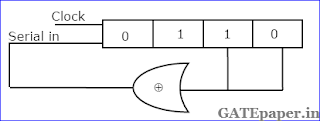### GATE 1992 ECE Video Solutions on Digital Circuits (Digital Electronics)

1. The logic realized by the circuit shown in figure is2. Choose the correct statement(s) from the following:
a. PROM contains a programmable AND array and a fixed OR array
b. PLA contains a fixed AND array and a programmable OR array
c. PROM contains a fixed AND array and a programmable OR array
d. PLA contains a programmable AND array and a programmable OR array

3. The figure shows the circuit of a gate in the Resistor Transistor Logic (RTL) family.The circuit represents a
a. NAND
b. AND
c. NOR
d. OR

4. The initial contents of the 4 bit serial in serial out, right shift, shift register shown in figure, are 0110. After three clock pulses are applied, the contents of the shift register will bea. 0000
b. 0101
c. 1010
d. 1111

5. In an 8085 microprocessor system with memory mapped I/O,
a. I/O devices have 16 bit addresses
b. I/O devices are accessed using IN and OUT instructions
c. There can be a maximum of 256 input devices and 256 output devices
d. Arithmetic and logic operations can be directly performed with the I/O data.
Solution:

6. The following program is run on 8085 microprocessor:At the completion of the execution of the program, the program counter of the 8085 contains _____________ and the stack pointer contains _______________.
Solution:

7. A new clocked X-Y flip flop is defined with two inputs, X and Y is in addition to the clock input. The flip flop functions as follows:
If XY = 00, the flip flop changes state with each clock pulse
If XY = 01, the flip flop state Q becomes ‘1’ with the next clock pulse
If XY = 10, the flip flop state Q becomes ‘0’ with the next clock pulse
If XY = 11, the change of state occurs with the clock pulse
a. Write the truth table for the XY flip flop
b. Write the excitation table for the XY flip flop
c. It is desirable to convert a J-K flip flop into X-Y flip flop by adding some external gates, if necessary. Draw a circuit to show how you will implement in X-Y flip flop using a J-K flip flop.

8. Dual slope integration type analog to digital converter provide
a. Higher speeds compared to all other types of A/D converters
b. Very good accuracy without putting extreme requirements on component stability
c. Good rejection of power supply hum
d. Better resolution compared to all other types of A/D converters for the same number of bits.

9. A combinational circuit has three inputs A, B and C and an output F. F is true only for the following combinations.
A is false and B is true
A is false and C is true
A, B and C are all false
A, B and C are all true
a. Write the truth table for F. Use convention, true = 1 and false = 0.
b. Write the simplified expression for F as a sum of products.
c. Write the simplified expression for F as a product of sums.
d. Draw the logic circuit implementation of F using the minimum number of 2 input NAND gates only.

10. Figure shows the memory circuit of 8085 microprocessor.a. What is the total size of the memory in the circuit?
b. What are the beginning and ending addresses of the memory in chip 1?
c. What are the beginning and ending addresses of the memory in chip 2?
d. Are the memory chips in the circuit ROM or RAM?
e. How will you replace the two NAND gates I the circuit with one 3 to 8 decoder without changing the memory size or the memory addresses? Assume that the decoder has one active high enable E1 and one active low enable E2.

1.Gate entry systems This particular is usually apparently essential and moreover outstanding truth along with for sure fair-minded and moreover admittedly useful My business is looking to find in advance designed for this specific useful stuffs…

2.A round of applause for your mind blowing article. Much thanks to you. Fantastic.

Name

Email *

Message *